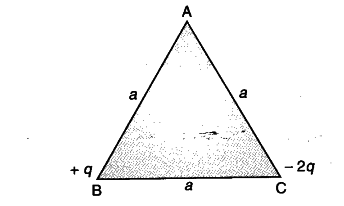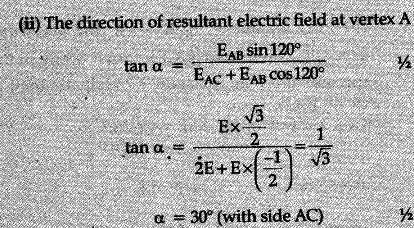# Two point charges + q and -2q are placed at the vertices 'B' and ‘C of an equilateral triangle ABC of side 'a' as given in the figure. Obtain the expression for

Two point charges + q and -2q are placed at the vertices ‘B’ and ‘C of an equilateral triangle ABC of side ‘a’ as given in the figure. Obtain the expression for (i) the magnitude and (ii) the direction of the resultant electric field at the vertex A due to these two charges.